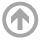Mathematics DepartmentMathematics with Economics Major

The mathematics with economics (SME) major, formerly called the quantitative economics (SQE) major, is an interdisciplinary mathematics and economics major administered by the mathematics department.  The goal of this major is to produce graduates who are adept at using mathematics to understand and evaluate problems in economics.  The curriculum consists of an equal number of advanced courses in mathematics and economics.  The mathematics with economics major prepares midshipmen with the analytic and computational tools to pursue further study in economics, finance, business administration or operations analysis -- disciplines that are all becoming increasingly mathematical.  In the Navy or Marine Corps, a graduates from this major would be well equipped to solve problems in areas such as weapons analysis, manpower studies, decision analysis,and strategic problems. The mathematics with economics major is a good choice for midshipmen who are fascinated by economic applications and comfortable with mathematical theory and computation.

Math with Economics Matrices

• SME Mathematics with Economics

Learning Outcomes

SME majors will be able to:

1. Speak, read and write several mathematical languages
2. Understand basic ideas of microeconomic and macroeconomic theory and apply them to economic problems, issues and policies.
3. Analyze economic models using appropriate mathematical languages.
4. Interpret economic models using appropriate mathematical languages
5. Collect and analyze data using appropriate mathematical languages to address empirical economic questions.